# ₦airaland Forum

Welcome, Guest: Join Nairaland / LOGIN! / Trending / Recent / New
Stats: 2,209,487 members, 4,821,818 topics. Date: Monday, 18 March 2019 at 10:59 PM

## What Is The Total Weights Of The Animals In This Picture? - Education (3) - Nairaland

(1) (2) (3) (4) (Reply) (Go Down)

 Re: What Is The Total Weights Of The Animals In This Picture? by phemmyfour: 2:00pm On Feb 13, 2015 a+b=10........eq1a+c=20.........eq2b+c=24.........eq3Combine eq2 and eq3Therefore b-a=4.......eq4Now, combine eq1 and eq4Therefore 2b = 14b=7a=3c=17Combine weight 7+3+17=27kg.......lobatan! Re: What Is The Total Weights Of The Animals In This Picture? by drey076(m): 2:02pm On Feb 13, 2015 husseinjnr:Let A= Rabbit B= Cat C = dogFrom Figure above, in simple analysis A + B = 10kg -----------equation 1A + C = 20kg -----------equation 2B + C = 24 kg ---------- equation 3From equation 2, A= 20- CFrom equation 3B= 24- CSubstitute A and B into equation 120-C + 24-C =1044-2C = 10-2C= 10-44-2C = -34C= 34/2C= 17Substitute C into equation 2A+ 17 = 20A= 3Substitute C into equation 3B+17 = 24B= 7ThereforeA= 3, B= 7, C= 17Total weight is 3+7+17 = 27Rabbit= 3kgCat = 7kgDog = 17kg good 1 Like Re: What Is The Total Weights Of The Animals In This Picture? by Nobody: 2:02pm On Feb 13, 2015 Let D = Weight of the dog, C = Weight of the cat, and R = Weight of the rabbitLet X = their combined weight.Required:X = D + C + RSolution:We are given the following:C + R = 10..............(1)C + D = 24..............(2)D + R = 20..............(3)From (1) we have the following:C = 10 - R..............(4)Substituting (4) in (2) we have:(10 - R) + D = 24===>> D - R = 24 - 10===>> D - R = 14...........(5)Solving (3) and (5) simultaneously, we have the following:D + R = 20.............(2)D - R = 14.............(3)Adding (2) and (3) we will have:(D + D) + (R - R) = (20 +14)====>> 2D = 34Dividing through by 2 we have:D = 17...............(6)Substitute for D in (2) we have:17 + R = 20 ===>> R = 20 - 17Hence, R = 3........(7)Substituting for R in (1) we have:C + 3 = 10 ===>> C = 10 -3 = 7.........(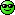Given that the combined weight is thus:X = D + C + RWe have from (6), (7) and (the following:X = 17 + 7 + 3===>> X = 27Therefore their combined weight is 27 kg 1 Like Re: What Is The Total Weights Of The Animals In This Picture? by Nobody: 2:07pm On Feb 13, 2015 Set theory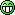Re: What Is The Total Weights Of The Animals In This Picture? by Nobody: 2:08pm On Feb 13, 2015 Folksyharry:what will the three animals weigh?Straight forward without all this mathematics; use the 2nd picture and d 3rd picture to demystify d mystery. Simple27kg The dog is 17kg Cat is 7kgAnd d Rabbit is 3kg Re: What Is The Total Weights Of The Animals In This Picture? by Youpele52: 2:20pm On Feb 13, 2015 This is how I did mine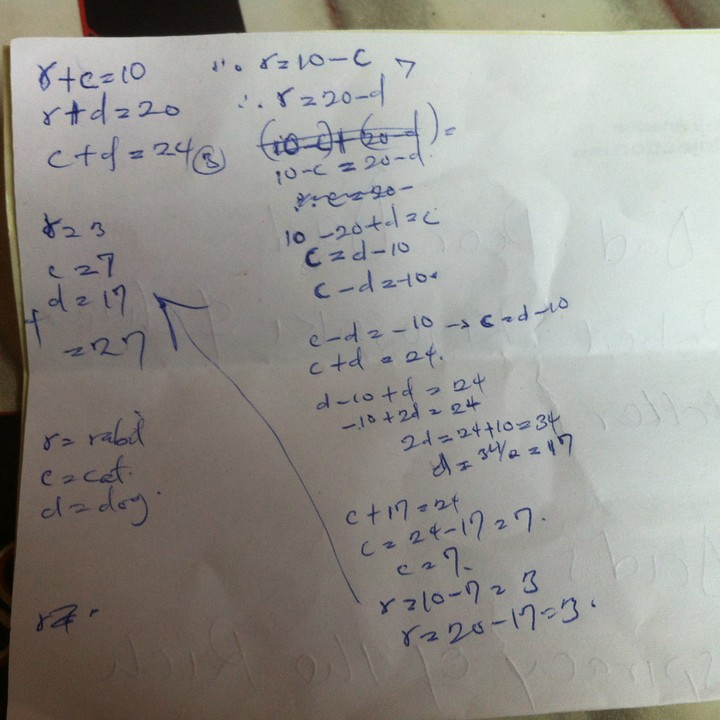Re: What Is The Total Weights Of The Animals In This Picture? by musicwriter(m): 2:22pm On Feb 13, 2015 What this maths question also show is Nairalanders can lie!. So, becausesomeone answered correctly, everybody now get the same answer?. No singlewrong answer?.If a Nairalander tell you to COME you'd better RUN. Re: What Is The Total Weights Of The Animals In This Picture? by nanizle(m): 2:27pm On Feb 13, 2015 No need for long calculations.Simply add up the weights (10+20+24)That's 54kg (made up of 2 cats, two dogs and two rabbits)54/2 = 27kg (1cat, 1dog and 1 rabbit) 5 Likes Re: What Is The Total Weights Of The Animals In This Picture? by teemy(m): 2:32pm On Feb 13, 2015 I did a simple figure 1,2,3 added together gives 54kg = combined weight of 2 rabbits + 2 cats + 2 dogs. Divided by 2 gives 27kg as answered by the algeraens. Re: What Is The Total Weights Of The Animals In This Picture? by kilokeys(m): 2:34pm On Feb 13, 2015 dechandel:When i tell you to apply logical reasoning in life.. I know what i am sayingImagine when a law student beat an engineering graduate in a maths essayif i catch u. u wanna start this engineering / law duel?parralel turning, broaching and drilling vs u yelling my name as i land my gavel in ur supreme immaculate court Re: What Is The Total Weights Of The Animals In This Picture? by palabest: 2:35pm On Feb 13, 2015 lifestyle1: Lets assume rabbit, cat and dog’s weight are R,C and D correspondingly.then we can sayEquation 1: R + C = 10Equation 2: D + R = 20Equation 3: C + D= 24Then we need to find value of C + R + DOn adding Equation 1,2 and 32R + 2 C + 2D = 54 => R+C+D = 27Na expo i use oThe most brilliant of all the solutions!!!! Re: What Is The Total Weights Of The Animals In This Picture? by aycd44(m): 2:39pm On Feb 13, 2015 27kg Re: What Is The Total Weights Of The Animals In This Picture? by Alexbond(m): 2:47pm On Feb 13, 2015 d total weight of d animals in d "picture" is equivalent to d weight of d picture. no calculations required :Pd total weight of d animals in d "picture" is equivalent to d weight of d picture. no calculations required Re: What Is The Total Weights Of The Animals In This Picture? by Nobody: 2:53pm On Feb 13, 2015 bros e never reach to comot CV nahusseinjnr:Let A= Rabbit B= Cat C = dogFrom Figure above, in simple analysis A + B = 10kg -----------equation 1A + C = 20kg -----------equation 2B + C = 24 kg ---------- equation 3From equation 2, A= 20- CFrom equation 3B= 24- CSubstitute A and B into equation 120-C + 24-C =1044-2C = 10-2C= 10-44-2C = -34C= 34/2C= 17Substitute C into equation 2A+ 17 = 20A= 3Substitute C into equation 3B+17 = 24B= 7ThereforeA= 3, B= 7, C= 17Total weight is 3+7+17 = 27Rabbit= 3kgCat = 7kgDog = 17kg 2 Likes Re: What Is The Total Weights Of The Animals In This Picture? by Hysmady(m): 2:54pm On Feb 13, 2015 Misogynist2014: That's how they fail exams. Who? Ur fellows? Re: What Is The Total Weights Of The Animals In This Picture? by Tonei: 2:59pm On Feb 13, 2015 Jesus is the answerRe: What Is The Total Weights Of The Animals In This Picture? by Misogynist2014(m): 3:01pm On Feb 13, 2015 Hysmady: Who? Ur fellows? He asked for collective weight, not individual weight. Re: What Is The Total Weights Of The Animals In This Picture? by Usman212s: 3:06pm On Feb 13, 2015 C + R=10kgD + R= 20kgD + C= 24kgAdding equation 1,2&32C+2D+2R =10+20+24C+D+R = 54/2 = 27kg Re: What Is The Total Weights Of The Animals In This Picture? by Oiselenjakhian(m): 3:11pm On Feb 13, 2015 Hello I am a web developer. My personal website is http://trustondevcenary.com please visit my site and fill the contact form if you want a website. Re: What Is The Total Weights Of The Animals In This Picture? by aniagoluaustin(m): 3:13pm On Feb 13, 2015 30 Re: What Is The Total Weights Of The Animals In This Picture? by MistadeRegal(m): 3:19pm On Feb 13, 2015 jacksparrow1207:YOU DULL SMALL SHA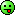Im com bright after im dull finish Re: What Is The Total Weights Of The Animals In This Picture? by Nobody: 3:32pm On Feb 13, 2015 MistadeRegal:Im com bright after im dull finish[size=18pt]THAT KIND CANDLE LIGHT BRIGHT [/size]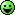Re: What Is The Total Weights Of The Animals In This Picture? by Timileyin(m): 3:35pm On Feb 13, 2015 omo, i have been calculation all day its 27 i think joor Re: What Is The Total Weights Of The Animals In This Picture? by follygabby: 3:39pm On Feb 13, 2015 since 99% of you said 27, so why do i stress myself to calculate it.? abeg d answer is 27 Re: What Is The Total Weights Of The Animals In This Picture? by Agbalanze(m): 3:39pm On Feb 13, 2015 Let cat be CLet dog be DLet Rabbit be RC+R=10..... EQU1D+R=20..... EQU2D+C=24..... EQU3D+C+R=?C=10-R.... EQU4D=20-R.... EQU5SUBSTITUTE EQU4 AND EQU5 INTO EQU3D+C=2420-R+10-R=2430-2R=24-2R=24-30-2R=-62R=6R=6/2R=3 (Rabbit)SUBSTITUTE R INTO EQU1C+3= 10C=10-3=7C=7 (Cat)SUBSTITUTE R INTO EQU2D+R=20D+3=20D=20-3D=17 (Dog)TOTAL= D+C+R= 17+7+3= 27kg Re: What Is The Total Weights Of The Animals In This Picture? by femi4love(m): 3:47pm On Feb 13, 2015 27 Re: What Is The Total Weights Of The Animals In This Picture? by serege(m): 3:55pm On Feb 13, 2015 kilokeys:lol.. i stumbled on this q last week.. in d dead of d night. went tthrough stress solving it.. while my e-bae dechandel made a good guess using a shorter method.. i almost cried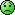oboy see kakulation.menn u try oRe: What Is The Total Weights Of The Animals In This Picture? by Nobody: 3:57pm On Feb 13, 2015 Yungwizzzy:How are mine supposed to know when it's just a drawing?bring the animals in real life and I'll weigh them simple!!1 Like Re: What Is The Total Weights Of The Animals In This Picture? by Hawlahscho(m): 4:21pm On Feb 13, 2015 kilokeys:lol.. i stumbled on this q last week.. in d dead of d night. went tthrough stress solving it.. while my e-bae dechandel made a good guess using a shorter method.. i almost criedNa ur handwriting be that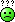Re: What Is The Total Weights Of The Animals In This Picture? by jasonkid007: 4:35pm On Feb 13, 2015 make dem enter soup pot first.ehen i go come siddon weigh them wella Re: What Is The Total Weights Of The Animals In This Picture? by Henrolla(m): 4:36pm On Feb 13, 2015 husseinjnr:Let A= Rabbit B= Cat C = dogFrom Figure above, in simple analysis A + B = 10kg -----------equation 1A + C = 20kg -----------equation 2B + C = 24 kg ---------- equation 3From equation 2, A= 20- CFrom equation 3B= 24- CSubstitute A and B into equation 120-C + 24-C =1044-2C = 10-2C= 10-44-2C = -34C= 34/2C= 17Substitute C into equation 2A+ 17 = 20A= 3Substitute C into equation 3B+17 = 24B= 7ThereforeA= 3, B= 7, C= 17Total weight is 3+7+17 = 27Rabbit= 3kgCat = 7kgDog = 17kgu sabi book o, thank God for people like u on NL Re: What Is The Total Weights Of The Animals In This Picture? by BMZK: 4:38pm On Feb 13, 2015 Visual method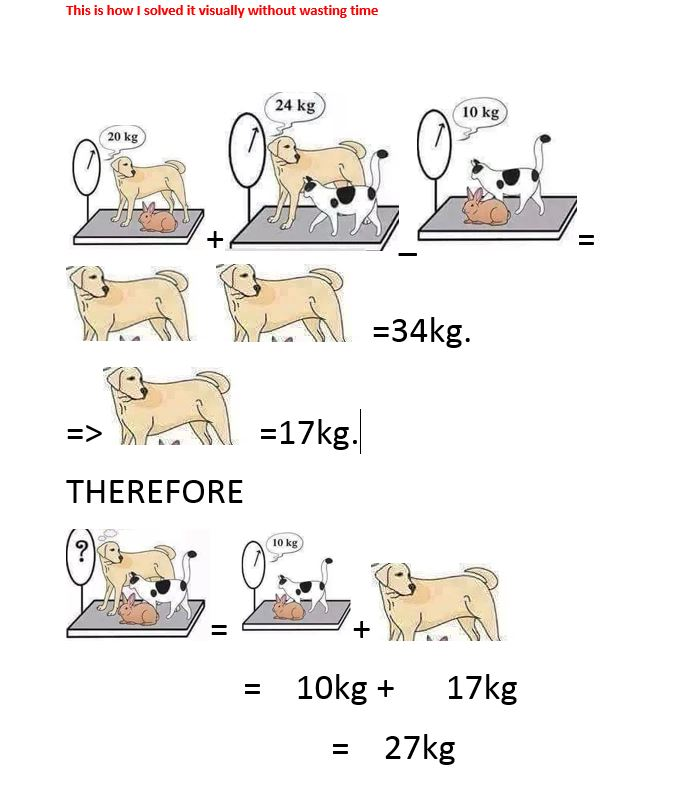(1) (2) (3) (4) (Reply)

(Go Up)

 Sections: politics (1) business autos (1) jobs (1) career education (1) romance computers phones travel sports fashion health religion celebs tv-movies music-radio literature webmasters programming techmarket Nairaland - Copyright © 2005 - 2019 Oluwaseun Osewa. All rights reserved. See How To Advertise. 102Disclaimer: Every Nairaland member is solely responsible for anything that he/she posts or uploads on Nairaland.